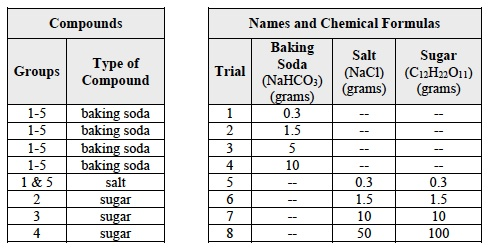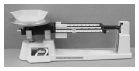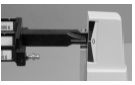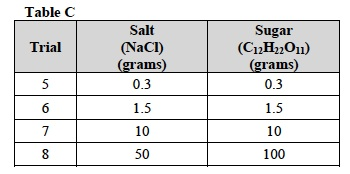# Investigation 1 – Lab### MINDSET

This Investigation is designed to:

• allow you to investigate how different compounds have different solubilities,
• familiarize you with making solutions consisting of a solid solute and a liquid solvent, and
• give you the opportunity to differentiate between a dissolved and undissolved solute.

### BE PREPARED

Student Preparation for the Investigation includes having students gather the following materials. This preparation takes place on lab day after student lab groups have settled at their assigned lab tables.

Note: The materials are listed in students’ SDRs. They are also listed below for your reference.

• (1) triple beam balance
• (4) 400ml beakers
• (4) glass stir rods
• (2) weigh dishes
• (1) lab scoop
• (1) 100 ml graduated cylinder
• (1) liter pitcher filled with water
• (1) stopwatch
• (1) roll of masking tape
• (1) marker
• gloves for each student
• goggles for each student
• approximately 20 g baking soda
• 70 g salt or 120 g sugar (see the table below)Direct one student from each lab group to collect the materials listed in their SDRs.

### INVESTIGATE

• You will make solutions by dissolving several different solid solutes in a liquid solvent, water.
• In Trials 1-4, each student group will make solutions using 4 different masses of baking soda, (NaHCO3).
• In Trials 5-8, each student group will be assigned to make solutions using 4 different masses of one of the following compounds: salt (NaCl) and sugar (C12H22O11).
• If necessary, review the triple beam balance and its use by using the procedures for Triple Beam Balance Equilibration and Triple Beam Balance Use and Operation in your Procedural Toolbox.
• Reflect on the PreLab video as you move through the procedural steps.
• During the Experiment, every procedural step is important. If one step is skipped, data can become invalid. To help you keep on track, read each step thoroughly, complete the step, then check it off (Read it – Do it – Check it).
• Complete all of the procedural steps in your SDR.

Note: The procedural steps are listed below for your reference.

The Experiment includes the following:

1. During Trials 1-4, each group will test the solubility of four different masses of baking soda.
1. To do this, each group will measure the mass of baking soda (NaHCO3) needed for each trial.
2. Then each group will try to dissolve the sample of baking soda (NaHCO3) in water.
3. Use the information in Table A for Trials 1-4.

Trial 1:

1. In this Trial, you will test the solubility of a 0.3 g sample of baking soda (NaHCO3) in water.
2. Measuring Mass Using a Weigh Dish or Beaker
1. How many grams of the compound should you use in Trial 1? Refer to Table A and record the mass. 0.3 g
2. Determine the mass of the weighing dish.
3. Place the weigh dish on the platform of the triple beam balance.1. Move the poises until the indicator line and center graduation line up.1. Record the mass of the weighing dish. 3.8 g
2. Adjust the poises so that the total is equal to the mass of the weighing dish plus the mass of the substance you wish to obtain. Find where the poises on the triple beam balance should be set to obtain the mass of the sample.
3. Add 0.3 g (the mass of the sample) to the mass of the weighing dish.
4. What should the combined mass of the weighing dish and the sample equal? 4.1 g
5. Move the poises to equal the combined mass.
6. Use the lab scoop to add the sample to the weigh dish until the poises are balanced.
7. When the balance indicator mark is lined up with the center balance mark, 0.3 grams of baking soda has been measured.
1. Pour the sample of the compound into one 400 ml beaker.
2. Use the graduated cylinder to measure 100 ml of water.
3. Pour the water into the beaker with the sample.
4. Stir the contents of the beaker with the stir rod for two minutes. Use the stopwatch to time the stirring.
5. After two minutes, observe the contents of the beaker. What do you observe? The baking soda (NaHCO3) is no longer visible. The liquid in the beaker is clear.
6. Is the sample soluble in 100 ml of water? Record your answer in the last column of Table A.

Trials 2-4:

1. In Trials 2-4 you will test the solubility of three other samples of baking soda (NaHCO3).
2. Use Table A to find out how many samples to use for each Trial.
3. Use masking tape to label three 400ml beakers as follows: Trial 2: 1.5g, Trial 3: 5.0g, Trial 4: 10.0g.
4. Record: Write the mass of the weighing dish in the first column of Table B.
5. Record: Write where the poises should be set for each mass in the last column of Table B.
6. Obtain the mass of each sample using the triple beam balance. Refer to Measuring Mass Using a Weigh Dish or Beaker and steps 2a through 2k of Trial 1 for help.
7. Test the solubility of each sample. Follow the steps below.
1. Pour the 1.5g sample into the 400ml beaker labeled Trial 2: 1.5g. Pour the other samples of the compound into the corresponding 400 ml beakers.
2. Use the graduated cylinder to measure 100 ml of water. Pour the water into the beaker for Trial 2 only.
3. Stir the contents of the beaker with the stir rod for two minutes. Use the stopwatch to time the stirring.
8. After you have stirred the liquid for two minutes, observe the contents of the beaker. What do you observe for the 1.5 g sample of baking soda (NaHCO3)?
9. Is the sample soluble in 100 ml of water? Record your answer in the last column of Table A.
10. For Trial 3, repeat steps 7a through 7c with the 5.0g sample.
11. After you have stirred the liquid for two minutes, observe the contents of the beaker. What do you observe for the 5.0g sample of baking soda (NaHCO3)?
12. Is the sample soluble in 100 ml of water? Record your answer in the last column of Table A.
13. For Trial 4, repeat steps 7a through 7c with the 10.0g sample.
14. After you have stirred the liquid for two minutes, observe the contents of the beaker. What do you observe for the 10.0g sample of baking soda (NaHCO3)?
15. Is the sample soluble in 100 ml of water? Record your answer in the last column of Table A.
16. Rinse out the beakers with water and remove the labels.

Trials 5-8:

1. In these Trials, you will test the solubility of one of the compounds in Table C.
2. In the first row of Table C, circle the compound that you will investigate.1. Using masking tape, label four 400 ml beakers with trial number and the number of grams of the compound for each trial.
2. Write the name of the compound above Table D. Obtain the mass of each sample using the triple beam balance. Use Table D and refer to Measuring Mass Using a Weight Dish or Beaker for help.
1. Record: Write the mass of the weighing dish in the first column of Table D.
2. Record: Write the mass of each sample in the middle column of Table D.
3. Record: Write where the poises should be set for each mass in the last column of Table D.

Trial 5:

1. Test the solubility of the sample for Trial 5. Follow the steps below.
1. Pour each sample of the compound into the 400 ml beaker labeled with the corresponding trial and mass.
2. Use the graduated cylinder to measure 100 ml of water. Pour the water into the beaker labeled Trial 5.
3. Stir the contents of the beaker with the stir rod for two minutes. Use the stopwatch to time the stirring.
2. After you have stirred the liquid for two minutes, observe the contents of the beaker. What do you observe for the first sample?
3. Is the sample soluble in 100 ml of water? Record your answer in the last column of Table E.

Trial 6:

1. For Trial 6, repeat steps 1a through 1c of Trial 5 with the next sample.
2. After you have stirred the liquid for two minutes, observe the contents of the beaker. What do you observe for this sample?
3. Is the sample soluble in 100 ml of water? Record your answer in the last column of Table E.

Trial 7:

1. For Trial 7, repeat steps 1a through 1c of Trial 5 with the next sample.
2. After you have stirred the liquid for two minutes, observe the contents of the beaker. What do you observe for this sample?
3. Is the sample soluble in 100 ml of water? Record your answer in the last column of Table E.

Trial 8:

1. For Trial 8, repeat steps 1a through 1c of Trial 5 with the next sample.
2. After you have stirred the liquid for two minutes, observe the contents of the beaker. What do you observe for this sample?
3. Was each sample of your compound soluble in 100 ml of water?
1. Share your data with the class.
2. Record: Complete Table F by filling in the white boxes with yes or no. Student answers may vary slightly based on their observations of the solutions. Sample data is provided.

### CLEAN UP

Be sure to clean up your lab bench after your experiments.